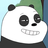# JR1024

V2EX 第 267288 号会员，加入于 2017-11-15 19:36:31 +08:00根据 JR1024 的设置，主题列表被隐藏

JR1024 最近回复了
 2021-04-01 11:19:34 +08:00

 2021-03-16 10:06:43 +08:00 回复了 Cbdy 创建的主题 › Java › JDK16 今日（2021.3.16）发布
1.6 打卡
 2021-01-13 09:55:37 +08:00 回复了 codingKingKong 创建的主题 › 分享创造 › 迫于小学生算术题, 连加连减生成可以用了

5+4+1= 7+0+1= 0+5+3= 0+1+1= 3+1+5=
1+3+4= 2+3+0= 8+2+0= 8+0+1= 10+0+0=
1+1+1= 3+3+4= 4+2+0= 4+6+0= 5+4+0=
5+3+2= 5+2+2= 5+0+1= 0+7+2= 3+6+0=
2+1+4= 1+2+3= 1+1+0= 4+4+2= 0+8+0=
0+3+2= 0+2+7= 2+7+0= 1+3+2= 0+3+4=
4+0+2= 2+4+1= 0+2+5= 6+0+2= 3+7+0=
3+3+2= 0+1+2= 2+2+0= 6+3+1= 2+1+1=
4+1+4= 6+2+0= 5+1+2= 0+1+3= 1+2+2=
1+3+0= 2+5+0= 2+6+2= 3+0+0= 3+5+0=
 2020-12-15 10:41:26 +08:00 回复了 tgich 创建的主题 › 宽带症候群 › 刚办了电信宽带+电视，请大佬们来告诉我赚了还是亏了

 2020-12-10 17:05:48 +08:00 回复了 rebnonu 创建的主题 › 推广 › 自家种的赣南脐橙剩最后两棵树 300 斤左右，抽奖 3 箱。

 2020-10-30 10:18:45 +08:00 回复了 wandehul 创建的主题 › 装修 › 一人一句装修的经验或者坑

 2020-05-08 17:13:05 +08:00

 2020-01-17 17:06:47 +08:00 回复了 gitbay 创建的主题 › 电影 › 迫于春节回家路上无聊，有什么好看的电影/电视剧推荐吗？

 2019-12-31 09:42:33 +08:00 回复了 zhuzhiqiang 创建的主题 › 程序员 › 你今天因为 YYYY-MM-dd 被提 BUG 了吗
Calendar calendar = Calendar.getInstance();
// 2019-12-31
calendar.set(2019, Calendar.DECEMBER, 31);
Date strDate1 = calendar.getTime();
// 2020-01-01
calendar.set(2020, Calendar.JANUARY, 1);
Date strDate2 = calendar.getTime();
// 大写 YYYY
DateFormat formatUpperCase = new SimpleDateFormat("YYYY/MM/dd");
System.out.println("2019-12-31 to YYYY/MM/dd: " + formatUpperCase.format(strDate1));
System.out.println("2020-01-01 to YYYY/MM/dd: " + formatUpperCase.format(strDate2));
// 小写 YYYY
DateFormat formatLowerCase = new SimpleDateFormat("yyyy/MM/dd");
System.out.println("2019-12-31 to yyyy/MM/dd: " + formatLowerCase.format(strDate1));
System.out.println("2020-01-01 to yyyy/MM/dd: " + formatLowerCase.format(strDate2));